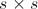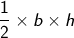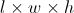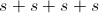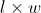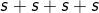# SSAT Elementary Level Math : How to find the perimeter of a square

## Example Questions

← Previous 1 3 4

### Example Question #1 : How To Find The Perimeter Of A Square

What is the perimeter of a square of area 36?Explanation:

The area of a square is the square of the length of its side (all sides are equal in a square). If the area is 36, then the length of each side is 6.

The perimeter of a square is 4 times the length of its side. Therefore, 4 times 6 is 24.

### Example Question #2 : How To Find The Perimeter Of A Square

Which of the following is NOT a quadrilateral?

trapezoid

nonagon

rhombus

parallelogram

nonagon

Explanation:

Quadrilaterals have 4 sides. A nonagon has 9 sides.

### Example Question #3 : How To Find The Perimeter Of A Square

What is the perimeter of a square that has a side measuring 3 inches in length?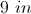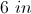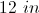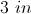Explanation:

Perimeter is the sum of all the sides. For a square,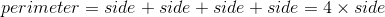.

Therefore, for this square,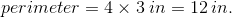### Example Question #4 : How To Find The Perimeter Of A Square

What is the perimeter of a square that has a side measuring 1 inch in length?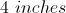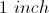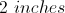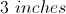Explanation:

Perimeter is the sum of all the sides. For a square,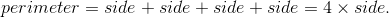Therefore, for this square,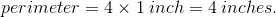### Example Question #5 : How To Find The Perimeter Of A SquareWhat is the perimeter of the square?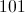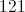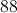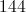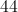Explanation:

To find the perimeter of this square, add all four sides together. Even though we are only given one side's length, every side of a square always has the same length!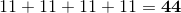### Example Question #6 : How To Find The Perimeter Of A SquareWhat is the perimeter of the square?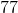Explanation:

To find the perimeter of a square, simply add all four sides together. Even though we are only given one side's length, squares always have four, equal sides!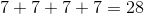### Example Question #7 : How To Find The Perimeter Of A Square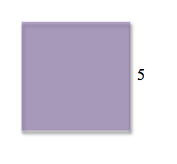What is the perimeter of the square?Explanation:

To find the perimeter of a square, add all four sides together. Since each side of a square is the same length, we only need one side's length to figure out the perimeter of a square!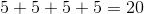### Example Question #8 : How To Find The Perimeter Of A Square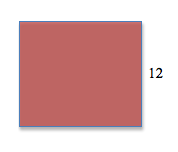What is the perimeter of the square?Explanation:

To find the perimeter of a square, simply add all four sides together. Since squares always have four equal sides, we only need the length of one side to determine the perimeter!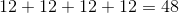### Example Question #9 : How To Find The Perimeter Of A Square

If John draws a square with a side of 7 centimeters, what is the square's perimeter?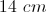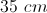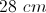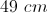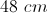Explanation:

To find the perimeter of a square, multiply the side length by 4: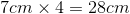Adding all four sides will produce the same result: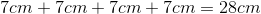### Example Question #10 : How To Find The Perimeter Of A Square

Which formula would you use to find the perimeter of a square?